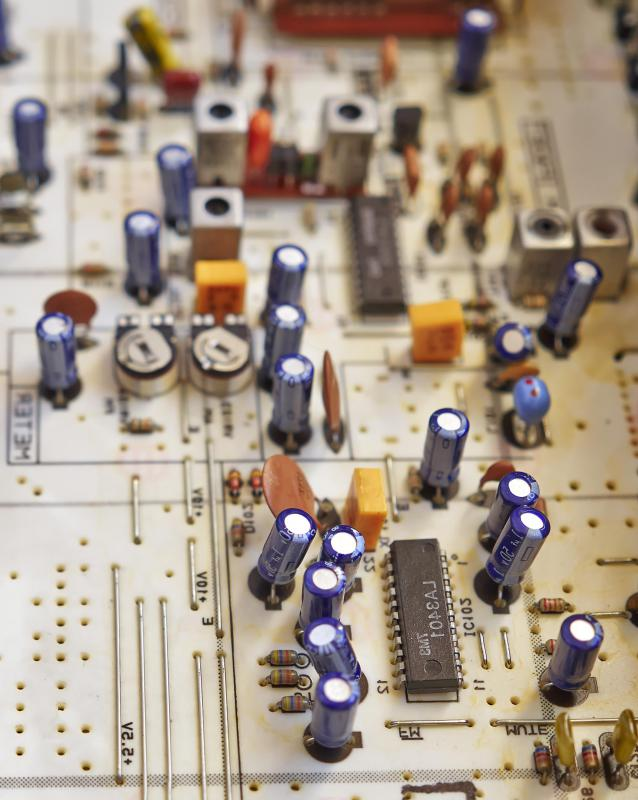# What Is a Resistor Network?

G.W. Poulos

A resistor network refers to a number of resistors configured into a given pattern. Most often, these networks use resistors connected end-to-end in series; however, a number of variations exist where the resistors are connected in parallel or series-parallel sequences resembling ladders. In all instances, the resistors in these networks act as voltage dividers, which divide the voltage applied to the circuit into smaller amounts. Practically, resistor networks are used to provide fractional supply voltages in various circuits or to perform digital-to-analog and analog-to-digital conversion functions.Carbon resistors are arranged in a pattern to form a resistor network.

Resistors are electronic components that resist the flow of electrical current by dissipating its voltage in a manner called dropping. Simply put, a resistor will drop a percentage of a circuit’s voltage. That percentage is equal to the value of a given resistor, in ohms, when compared to the circuit’s total resistance. For example, a 10-ohm resistor will drop 10% of the voltage in a circuit that has 100 ohms worth of resistance.A resistor is an electronic component that can lower a circuit’s voltage and its flow of electrical current.

If a resistor network has five 1-ohm resistors, placed in series, and a 5-volt power supply is connected, each of the five resistors would drop one-fifth of the 5 volts, or 1 volt each. A resistor network, in this way, can provide fractional power supply voltages to other circuits. Since the voltage drop across any one resistor is equal to that resistor’s value in ohms, when compared to the entire circuit’s resistance, practically any voltage desired that is less than the applied voltage is possible in a resistor network.

For example, if four resistors were connected in series, with three measuring 1 ohm and the fourth measuring 2 ohms, the total circuit resistance would be 5 ohms. While the three 1-ohm resistors will drop 1 volt each, the 2-ohm resistor will drop 2 volts. Connecting a circuit to that point in the resistor network will provide a 2-volt power source.

There are other uses for resistor networks. If instead of using the points between the resistors in the network to provide differing voltages, they are all used to provide the same voltage, the network can then be used to convert analog signals to digital information. This is accomplished by connecting a digital gate to each of the voltage points in the network. When an analog signal is applied, the dividing of the voltage will give a series of escalating high or low voltages, depending on the input signal, which the digital gates read as on or off. The gates will then send that information on to other circuits as ones or zeroes, converting the analog signal to digital information.

Resistors can also be configured in a series-parallel manner, called an R-2R network. In this configuration, digital gates inject high or low voltage representing ones and zeroes into the points between the resistors in the network. This causes the total voltage drop across the resistors in the network to vary proportionally to the overall input, instead of simply turning on and off with the individual digital inputs. Outputs from these sorts of networks are constantly varying analog signals created from digital inputs.

Resistor networks are heavily used in electronics. Though they are used for digital-to-analog and analog-to-digital conversion, they are more frequently used as simple voltage dividers for power functions. In this way, resistor networks help supply various voltages as needed to many different circuits in different devices.

## You might also Like